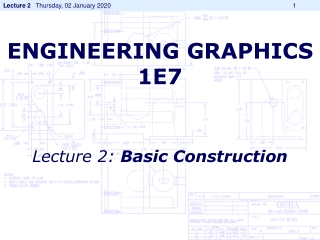DownloadDownload PresentationENGINEERING GRAPHICS 1E7

# ENGINEERING GRAPHICS 1E7

Download Presentation## ENGINEERING GRAPHICS 1E7

- - - - - - - - - - - - - - - - - - - - - - - - - - - E N D - - - - - - - - - - - - - - - - - - - - - - - - - - -
##### Presentation Transcript

1. ENGINEERING GRAPHICS1E7 Lecture 2: Basic Construction

2. DRAWING LINES Drawing Parallel Lines

3. DRAWING LINES Drawing Perpendicular Lines

4. A B DRAWING LINES Bisection of A Line • Place your compass point on A and stretch the compass MORE THAN half way to point B. • With this length, swing a large arc that will go BOTH above and below segment AB.  • Without changing the span on the compass, place the compass point on B and swing the arc again.  The new arc should intersect the previous one above and below the segment AB. • With your scale/ruler, connect the two points of intersection with a straight line. • This new straight line bisects segment AB.  Label the point where the new line and AB cross as C. • Segment AB has now been bisected and AC = CB.

5. DRAWING LINES Divide A Line into Multiple Sections • From one end of the given line AB (say, A) draw a line AC at a convenient angle • Using a scale/ruler divide the BC into the required number of parts making them of any suitable length. • Join the last point on line AC (say, C) to B • Draw construction lines through the other points on the line AB which are parallel to CB

6. DRAWING LINES Bisection of An Angle • Place the point of the compass on the vertex of angle BAC (point A). • Stretch the compass to any length so long as it stays ON the angle. • Swing an arc with the pencil that crosses both sides of angle ABC.  This will create two intersection points (E and F) with the sides of the angle. • Place the compass point on E, stretch your compass to a sufficient length and draw another arc inside the angle - you do not need to cross the sides of the angle. • Without changing the width of the compass, place the point of the compass on F and make a similar arc.  These two small arcs in the interior of the angle should be crossing each other. • Connect the point of intersection of the two small arcs to the vertex A of the angle with a straight line.

7. DRAWING LINES Find the Centre of an Arc • Select three points A, B and C on the arc and join AB and BC • Bisect AB and BC. • Fine the intersection point of the bisecting lines/bisectors. That is the centre of the arc.

8. DRAWING LINES Inscribe a Circle in a Triangle • Bisect angle ABC and angle BAC. • Fine the intersection point of the bisecting lines/bisectors. That is the centre of the circle. • The radius of the circle is the length of a perpendicular line on any of the sides of the triangle drawn from the centre of the circle.

9. DRAWING LINES Circumscribe a Circle on a Triangle • Bisect sides AC and BC. • Fine the intersection point of the bisecting lines/bisectors. That is the centre of the circle. • The radius of the circle is the length of a line joining any one of the vertices of the triangle to the centre of the circle.

10. DRAWING LINES Draw a Hexagon • To draw a regular hexagon given the distance across flats Draw a circle having a diameter equal to the distance across flats. • Draw tangents to this circle with a 60° set square to produce the hexagon.

11. DRAWING LINES Draw a Hexagon • To draw a regular hexagon given the distance across corners, draw a circle having a diameter equal to the distance across corners • Step off the radius round it to give six equally spaced points. • Join these points to form the hexagon.

12. Ellipse Construction Draw the axes AB and CD and draw circles (called auxiliary circles) on them as diameters. Divide the circles into a number of equal parts, by radial lines through O. Each of the radial lines intersect the major and minor auxiliary circle. Through the points where radial lines cut the major auxiliary circles drop vertical perpendiculars, and through the points where the radial lines cut the minor auxiliary circle draw horizontals to cut the verticals. These intersections are points on the ellipse.

13. CYCLOID • The cycloid is the locus of a point on the rim of a circle rolling along a straight line.

14. HYPOCYCLOID The curve produced by fixed point P on the circumference of a small circle of radius a rolling around the inside of a large circle of radius b.

15. EPICYCLOID The path traced out by a point P on the edge of a circle of radius a rolling on the outside of a circle of radius b.

16. A B C Involute of a line (AB):

17. What is an involute?? • Attach a string to a point on a curve. • Make the string a tangent to the curve at the point of attachment. • Then wind the string up, keeping it always taut. The locus of points traced out by the end of the string is called the involute of the original curve. • The original curve is called the evolute of its involute.

18. Example: Circle

19. Example: Triangle

20. Archimedean Spiral • Spiral of Archimedes is a spiral with polar equation

21. Try this!